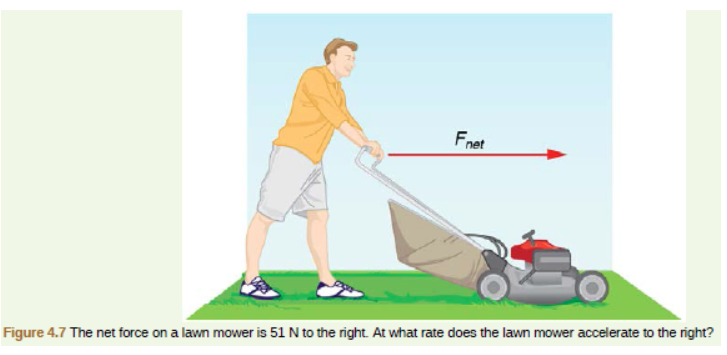# Problem: In Figure 4.7, the net external force on the 24-kg mower is stated to be 51 N. The force of friction opposing the motion in 24 N.  Suppose the mower is moving at 1.5 m/s when the force F is removed. How far will the mower go before stopping?

###### FREE Expert Solution

Uniform accelerated motion (UAM) equations:

Newton's second law:

$\overline{){\mathbf{\Sigma }}{\mathbf{F}}{\mathbf{=}}{\mathbf{m}}{\mathbf{a}}}$

ΣF = - f

a = - f/m = - 24/24 = - 1 m/s2

84% (130 ratings)###### Problem Details

In Figure 4.7, the net external force on the 24-kg mower is stated to be 51 N. The force of friction opposing the motion in 24 N.

Suppose the mower is moving at 1.5 m/s when the force F is removed. How far will the mower go before stopping?